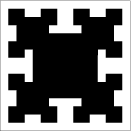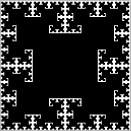Mathematical and Physical Journal
for High Schools
Issued by the MATFUND Foundation
 Already signed up? New to KöMaL?# Exercises and problems in InformaticsApril 2003

I. 49. N fans watching a match throw up their caps as their team wins and then every fan catches exactly one cap falling down. Write a program (i49.pas, ...) that displays the number of possible ways of exactly K (0$\displaystyle \le$K$\displaystyle \le$N) ones out of the N (0$\displaystyle \le$N$\displaystyle \le$12) fans getting their own caps back.

The example shows all cases for N,K$\displaystyle \le$4.
(10 points)

 K=0 K=1 K=2 K=3 K=4 N=0 1 N=1 0 1 N=2 1 0 1 N=3 2 3 0 1 N=4 9 8 6 0 1

I. 50. We make a wallpaper-pattern by first dividing a square into four equal squares, then removing the quarters adjacent to the centre of the original square. These steps are iteratively applied again to the remaining 3 quarters in each smaller square. We repeat the process N times, see the Figures.N=1 N=2 N=3 N=6

Your program (i50.pas, ...) should display the resulting wallpaper-pattern if N (1$\displaystyle \le$N$\displaystyle \le$10) is given.
(10 points)

I. 51. At the beginning of the day on a stock exchange, the current rate of each share is known. During the day we get a list of the actual sales (i.e. which and how many shares are sold as well as their sale prices) arranged in chronological order (at most 1000 sales are recorded). Columns of the first figure show the number of the transaction, the name of the share, the amount sold and the sale price, respectively.

 Time Share Amount Price 1 OTP 20 1500 2 OTP 23 1300 3 MALÉV 5 1000 4 OTP 20 1400 5 MOL 80 1000 6 MOL 10 900

Prepare your sheet (i51.xls) which - using these data - computes for each stock (i.e. columns of the second figure) its final price at the end of the day (second row), the difference (third row) between the starting price (given in the first row) and final price, the amount of stocks sold (fourth row) and the total value of that stock (fifth row). Stocks that were sold at a higher price on the whole compared to their daily starting prices should be marked red, while those at lower prices should be marked blue.
(10 points)

 OTP MÁV MALÉV MOL Starting price 1342 1443 1443 1111 Final price 1400 1443 1000 900 Difference 58 0 -443 -211 Amount 63 0 5 90 Total value 87900 0 5000 89000